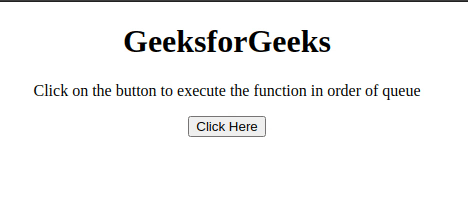Related Articles
How to store JavaScript functions in a queue and execute in that order?
• Last Updated : 15 Jul, 2020

The task is to execute the functions in the order defined in the queue with the help of JavaScript. There are two approaches that are discussed below.

Approach 1: Declare the functions and use array push() method to push the functions in the array. Later traverse the array and execute the functions one by one.

• Example: This example implements the above approach.

 ```<``html``>``    ``<``head``>``        ``<``title``>``            ``Store JavaScript functions in ``            ``a queue and execute in that order``        ````    ````    ``<``body` `style``=``"text-align: center;"``>``        ``<``h1``>``            ``GeeksforGeeks``        ````        ``<``p` `id``=``"GFG_UP"``>``        ``<``button` `onclick``=``"myGFG()"``>``            ``Click Here``        ````        ``<``p` `id``=``"GFG_DOWN"``>``        ``<``script``>``            ``var up = document.getElementById("GFG_UP");``            ``up.innerHTML = ``              ``"Click on the button to execute "+``              ``"the function in order of queue";``            ``var down = document.getElementById("GFG_DOWN");``            ``function myGFG() {``               ` `                ``// First function``                ``function function1() {``                    ``alert("First Function");``                ``}``               ` `                ``// Second function``                ``function function2() {``                    ``alert("Second Function");``                ``}``                ``var orderQueue = [];``               ` `                ``// Push them in queue``                ``orderQueue.push(function1);``                ``orderQueue.push(function2);``                ``while (orderQueue.length > 0) {``                    ``// Execute in order``                    ``orderQueue.shift()();``                ``}``                ``down.innerHTML = ``                  ``"Functions executed in queue order";``            ``}``        ````    `````
• Output:Approach 2:Declare the functions and use arrayindexing to assign the functions to index of the array in the order. Later traverse the array and execute the functions one by one.

• Example: This example implements the above approach.
 ```<``html``>``    ``<``head``>``        ``<``title``>``            ``Store JavaScript functions in a ``            ``queue and execute in that order``        ````    ````    ``<``body` `style``=``"text-align: center;"``>``        ``<``h1``>``            ``GeeksforGeeks``        ````        ``<``p` `id``=``"GFG_UP"``>``        ``<``button` `onclick``=``"myGFG()"``>``            ``Click Here``        ````        ``<``p` `id``=``"GFG_DOWN"``>``        ``<``script``>``            ``var up = document.getElementById("GFG_UP");``            ``up.innerHTML = ``              ``"Click on the button to execute "+``              ``"the function in order of queue";``            ``var down = document.getElementById("GFG_DOWN");``            ``function myGFG() {``               ` `                ``// First function``                ``function function1() {``                    ``alert("First Function");``                ``}``               ` `                ``// Second function``                ``function function2() {``                    ``alert("Second Function");``                ``}``                ``var functions = new Array();``                ``// Adding the functions in the order``                ``// in queue(array)``                ``functions = function1;``                ``functions = function2;``                ``for (var i = 0; i < ``functions.length``; i++) {``                   ` `                    ``// Executing them in order.``                    ``functions[i].call();``                ``}``                ``down.innerHTML` `= ``                    ``"Functions executed in queue order"``;``            ``}``        ````    `````
• Output:My Personal Notes arrow_drop_up